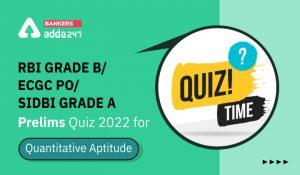Latest Banking jobs   »   Quantitative Aptitude Quiz 23 June- Daily...

# Quantitative Aptitude Quiz 23 June- Daily Quantitative Aptitude Quiz for SBI PO Prelims 2020

Adda 247 will provide you daily Quantitative Aptitude Mock of “SBI PO Prelims. In today’s Quantitative Aptitude Mock, we have Quadratic Inequalities. Stay tuned with bankersadda to get Quantitative Aptitude Mock and boost your preparation for the upcoming bank exam. You can also prepare with the study plan for SBI PO available on the bankersadda website.Directions (1-15): In following questions two equations are given. Solve the equations and give answer

Q1.(a) if x < y

(b) if x > y

(c) if x ≤ y

(d) if x ≥ y

(e) if x = y or no relation can be established

Q2.(a) if x < y

(b) if x > y

(c) if x ≤ y

(d) if x ≥ y

(e) if x = y or no relation can be established

Q3.(a) if x < y

(b) if x > y

(c) if x ≤ y

(d) if x ≥ y

(e) if x = y or no relation can be established

Q4.(a) if x < y

(b) if x > y

(c) if x ≤ y

(d) if x ≥ y

(e) if x = y or no relation can be established

Q5.(a) if x < y

(b) if x > y

(c) if x ≤ y

(d) if x ≥ y

(e) if x = y or no relation can be established

To attempt the complete quiz refer to the links given below:

Practice with Crash Course and Online Test Series for SBI PO Prelims:

#### If you are preparing for SBI PO Prelims Exam, then you can also check out a video for Quantitative Aptitude below:

Check updates related to notification, vacancies, eligibility, exam pattern, syllabus and more related to upcoming bank and insurance exams 2020:

#### Congratulations!Incorrect details? Fill the form again here

•Quantitative Aptitude Quiz For IDBI AM/E...
•Quantitative Aptitude Quiz For IDBI AM/E...
•Quantitative Aptitude Quiz For IDBI AM/E...
•Quantitative Aptitude Quiz For SBI Clerk...
•Quantitative Aptitude Quiz For RBI Grade...
•Quantitative Aptitude Quiz For SBI Clerk...